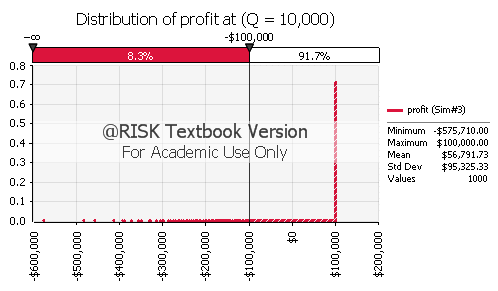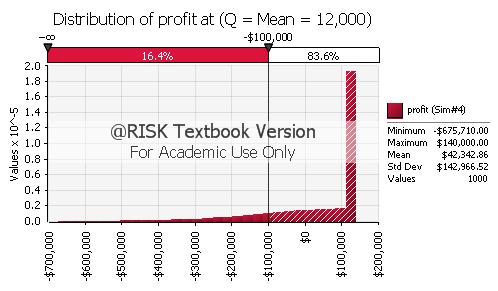0

# Simulation Modeling Using @Risk – Egress Inc. Ski Jacket Production CaseEmekaDec 23, 2011 | edited Jan 01, 2012 - by @Emeka

This is a homework on Data Analysis and Simulation Modeling. If you use the book: Data Analysis, Optimization, and Simulation Modeling by Albright, Zappe and Winston, you might have come across this question on page 985 of the book.

I did it and scored 100%, so I thought I should share it with others to give you insights on how to solve the problem.

#### Questions:

Assume that P is the price Egress charges retailers. Any ski jackets that do not sell in the season can be sold off by Egress on discount for V per jacket. F is the fixed cost of plant and equipment. This cost is incurred regardless of the size of the production run.

• Egress management thinks that a normal distribution is a likely model for the unknown demand in the coming year. What mean and standard deviation should Egress utlize for their demand
distribution? This question is basically asking you to fit a distribution to the data set.
• Simulate 1000 possible outcomes for demand in the
coming year. Based on these scenarios, what is the expected profit should Egress produce Q = 7800 ski jackets? What is the expected profit should Egress produce Q = 12,000 ski jackets? What is the standard deviation of profit in these two cases?
• How many ski jackets should Egress produce to maximize expected profit? Call this quantity Q.
• Should the Mean Demand equal Q? Give reasons
• Draw a histogram of profit at the production level Q and at when Q equals the Mean Demand and state the probability of a loss greater than 100,000 in both cases.

#### Solutions:

Estimated demands by 12 employees:

14,000   16,000
13,000   8,000
14,000   5,000
14,000   11,000
15,500   8,000
10,500   15,000

Monetary Values (Inputs):

Variable production cost per unit (C): \$80
Selling price per unit (S): \$100
Salvage value per unit (V): \$30
Fixed production cost (F): \$100,000

Formulars Used:

Revenue = S * IF(D < Q, D, Q)
Salvage value = V * IF(D < Q, Q – D, 0)
Profit = Revenue + Salvage value – Var cost – Fixed cost
Fixed Cost = F
=RiskSimtable(B37:F37)
=ROUND(RiskNormal(B21,B22),0)

Question (1):

Mean = 12,000
STD = 3,497

Therefore the demand D ~N(Mean=12,000, STD=3,497)
that is demand is normally distributed with mean 12000 and standard deviation 3497.

Question (2):

Quantity = 6,000

 Demand Revenue Variable Cost Fixed Cost Salvage Value Profit 13687 \$600,000 \$480,000 \$100,000 \$0 \$20,000

Simulation with 1000 iterations for the 12 expected demand values given a set of 5 chosen quantities:

 S/N 1 2 3 4 5 Quantity 6,000 8,000 10,000 12,000 7,800 Mean 15689.82 44604.76 56791.73 42342.86 42292.53 STD 27367.502 54962.06512 95325.33295 142966.523 51599.59005

At quantity 7,800, the Mean is 42292.53 and Standard deviation is 51599.59005 and at quantity 12,000, the Mean is 42342.86 and Standard deviation is 142966.523.

Question (3):

According to the simulation, the optimal quantity (Q) that Egress should produce is 10,000 where the average profit 56791.73 is at the highest.

Question (4):

To find out if Q = 10,000 should equal mean demand = 12,000,
average and standard deviation of profits at the two quantities (Q = 10,000 and Q=12,000) can be compared:

Difference in average profits between the two quantities:14448.87
Difference in STD of profits between the two quantities:47641.19008

The difference in average profits between the two quantities is quite significant, and it can also be shown by calculating the confidence interval that this difference is statistically significant.

The difference in their standard deviations removes Q = 12,000 with over 140,000 STD (high risk) out of the question,
making Q=10,000 the logical choice.

Question (5):This histogram shows the profit distribution is highly skewed to the left.The probability of losses greater than \$100,000 is 0.083,that is losses greater than \$100,000 happen about 8% of the time.This histogram also shows the profit distribution is highly skewed to the left.The probability of losses greater than \$100,000 is 0.164,that is losses greater than \$100,000 happen about 16% of the time.

If you have questions leave a comment in the comment section below or use the email icon on the left side of your computer screen to email me.maki Sep 10, 2015
0

how did you do 2nd question on excel?

edited Sep 10, 2015 - by @maki28793

More from @Emeka